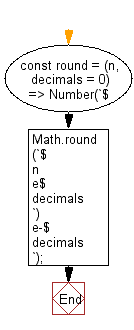# JavaScript: Round a number to a specified amount of digits

## JavaScript fundamental (ES6 Syntax): Exercise-152 with Solution

Write a JavaScript program to round a number to a specified amount of digits.

• Use Math.round() and template literals to round the number to the specified number of digits.
• Omit the second argument, decimals, to round to an integer.

Sample Solution:

JavaScript Code:

``````//#Source https://bit.ly/2neWfJ2
const round = (n, decimals = 0) => Number(`\${Math.round(`\${n}e\${decimals}`)}e-\${decimals}`);

console.log(round(1.005, 2));
console.log(round(1.05, 2));
console.log(round(1.0005, 2));
```
```

Sample Output:

```1.01
1.05
1
```

Pictorial Presentation:Flowchart:Live Demo:

See the Pen javascript-basic-exercise-152-1 by w3resource (@w3resource) on CodePen.

Improve this sample solution and post your code through Disqus

What is the difficulty level of this exercise?

Test your Programming skills with w3resource's quiz.

﻿